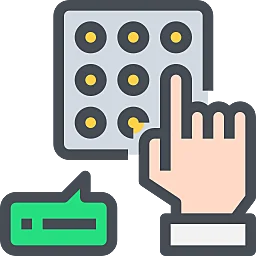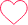# Numbers, Decimals And Fractions Tips, Tricks And Shortcuts

## Shortcuts for Number, Decimals, and Fractions

Here are some Tips, tricks and shortcuts For Numbers Decimals Fractions which will directly help in different competitive and recruitment exams.

As we know that numbers, decimals and fractions is a very basic topic and there are multiple methods to solve them.## Tips and Tricks for Decimal and Fraction

### Tips For Numbers Decimals Fractions

1) $(a – b)^{2} = (a^{2} + b^{2} – 2ab)$
2) $(a + b)^{2} = (a^{2} + b^{2} + 2ab)$
3) $(a + b) (a – b) = (a^{2} – b^{2} )$
4) $(a^{3} + b^{3}) = (a + b) (a^{2} – ab + b^{2})$
5) $(a^{3} – b^{3}) = (a – b) (a^{2} – ab + b^{2})$
6) $(a + b + c)^{2} = a^{2} + b^{2} + c^{2} + 2 (ab + bc + ca)$
7) $(a^{3} + b^{3} + c^{3} – 3abc) = (a + b + c) (a^{2}+ b^{2} + c^{2} – ab – bc – ac)$

### Tricks and shortcuts for Decimal and fraction

1. Decimal Fractions:
Fractions in which denominators are powers of 10 are known as decimal fractions.
Thus, 1/10 = 1 tenth = .1; 1/100 = 1 hundredth = .01;
88/100 = 88 hundredths = .88; 6/1000 = 6 thousandths = .006, etc.;

2. Conversion of a Decimal into Vulgar Fraction:
Put 1 in the denominator under the decimal point and annex with it as many zeros as is the number of digits after the decimal point. Now, remove the decimal point and reduce the fraction to its lowest terms.
Thus, 0.25 = 25/100 = 1/4 ; 2.008 = 2008/1000 = 251/125.

3. Annexing Zeros and Removing Decimal Signs:
Annexing zeros to the extreme right of a decimal fraction does not change its value. Thus, 0.8 = 0.80 = 0.800, etc.

If numerator and denominator of a fraction contain the same number of decimal places, then we remove the decimal sign.

Thus, 1.84/2.99 = 184/299 = 8/13.

4. Operations on Decimal Fractions:

i. Addition and Subtraction of Decimal Fractions: The given numbers are so placed under each other that the decimal points lie in one column. The numbers so arranged can now be added or subtracted in the usual way.

ii. Multiplication of a Decimal Fraction By a Power of 10: Shift the decimal point to the right by as many places as is the power of 10.
Thus, 5.9632 x 100 = 596.32; 0.073 x 10000 = 730.

iii. Multiplication of Decimal Fractions: Multiply the given numbers considering them without decimal point. Now, in the product, the decimal point is marked off to obtain as many places of decimal as is the sum of the number of decimal places in the given numbers.
Suppose we have to find the product (.2 x 0.02 x .002).
Now, 2 x 2 x 2 = 8. Sum of decimal places = (1 + 2 + 3) = 6.
.2 x .02 x .002 = .000008

iv. Dividing a Decimal Fraction By a Counting Number: Divide the given number without considering the decimal point, by the given counting number. Now, in the quotient, put the decimal point to give as many places of decimal as there are in the dividend.

Suppose we have to find the quotient (0.0204 Ã• 17). Now, 204 Ã• 17 = 12.

Dividend contains 4 places of decimal. So, 0.0204 Ã• 17 = 0.0012

v. Dividing a Decimal Fraction By a Decimal Fraction: Multiply both the dividend and the divisor by a suitable power of 10 to make divisor a whole number.

Now, proceed as above.

Thus, 0.00066/0.11 = 0.00066 x 100/0.11 x 100 = 0.066/11 = .006

5. Comparison of Fractions:
Suppose some fractions are to be arranged in ascending or descending order of magnitude, then convert each one of the given fractions in the decimal form, and arrange them accordingly.
Let us to arrange the fractions 3/5, 6/7 and 7/9 in descending order.

Now, 3/5 = 0.6, 6/7 = 0.857, 7/9 = 0.777…

Since, 0.857 > 0.777… > 0.6. So, 6/7 > 7/9 > 3/5 .

6. Recurring Decimal:
If in a decimal fraction, a figure or a set of figures is repeated continuously, then such a number is called a recurring decimal.

If a single figure is repeated, then it is expressed by putting a dot on it. If a set of figures is repeated, it is expressed by putting a bar on the set.

Thus, 1/3 = 0.333… = 0.3; 22/7 = 3.142857142857…. = 3.142857.

Pure Recurring Decimal: A decimal fraction, in which all the figures after the decimal point are repeated, is called a pure recurring decimal.

Converting a Pure Recurring Decimal into Vulgar Fraction: Write the repeated figures only once in the numerator and take as many nines in the denominator as is the number of repeating figures.
Thus, 0.5 = 5/9; 0.53 = 53/99; 0.067 = 67/999, etc.

Mixed Recurring Decimal: A decimal fraction in which some figures do not repeat and some of them are repeated, is called a mixed recurring decimal.
Eg. 0.1733333.. = 0.173.

Now let us look at some of the Tips For Numbers Decimals Fractions through the given examples.

Question 1: Evaluate $\frac{(3.8^2-1.2^2)}{(3.8 -{1.2})}\$

(a) 5.2
(b) 4.8
(c) 4
(d) 5

Explanation :

$\frac{(3.8^{2}-1.2^{2})}{(3.8 -{1.2})}\$
$\frac {(3.8 + 1.2)( 3.8 – 1.2)} {( 3.8 – 1.2 )}$

= 3.8+1.2 =5

Question 2: If 1.5x = 0.04y, then the value of $\frac { (y – x)}{ (y+x) }$ is :

(a) 73/77
(b) 7.3/77
(c) 730/77
(d) 7300/77

Explanation:

$\frac{ (x)}{ (y) }$

= $\frac{ (0.04)}{ (1.5) }$

= $\frac{ (4)}{ (150) }$

= $\frac{ (2)}{ (75) }$

= > $\frac{ (y – x)}{ (y+x) }$

= $\frac{(1 – \frac{x}{y})} {( 1 + \frac{x}{y})}$

= $\frac {1 – \frac{2}{75}}{1 – \frac{2}{75}}$

= 73/77

Question 3: If 47. 2506 = 4*A + 7/B + 2*C + 5/D + 6*E, then the value of 5*A + 3*B + 6*C + D + 3*E is :

(a) 53.6003
(b) 53.603
(c) 153.6003
(d) 213.003

Explanation:

4*A + 7/B + 2*C + 5/D + 6*E =47.2506
=> 4 * A + 7/B + 2 *C + 5/D + 6*E = 40 + 7 + 0.2 + 0.05 + 0.0006
Comparing the terms on both sides, we get :
4*A =40, 7/B = 7, 2*C = 0.2, 5/D = 0.05, 6*E = 0.0006
A = 10,
B = 1,
C = 0.1,
D = 100,
E = 0.0001.
5*A + 3*B + 6*C + D + 3*E =(5*10) + (3*1) + (6*0.1) +100 +(3*0.0001)
=50 + 3 + 0.6 + 100 + 0.003 = 153.6003.

Question 4 : A recipe requires 3/4 cup of sugar to make 12 cookies. How much sugar would be needed to make 36 cookies?

A. 2.25
B. 2.5
C. 3.5
D. 4.5

Solution and Explanation:
To find the amount of sugar needed to make 36 cookies, we can set up a proportion based on the given information:

Sugar needed / Number of cookies = 3/4 cup / 12 cookies

Let’s solve for the unknown, which is the amount of sugar needed for 36 cookies:

Sugar needed / 36 = (3/4) cup / 12

Cross-multiplying gives us:

Sugar needed * 12 = 36 * (3/4)

Sugar needed * 12 = 108/4

Simplifying the right side:

Sugar needed * 12 = 27

Dividing both sides by 12:

Sugar needed = 27 / 12

Sugar needed = 2.25 cups

Therefore, to make 36 cookies, you would need 2.25 cups of sugar

Question 5: Evaluate 1082 using (a + b)2 formula.

A. 11645
B. 12547
C. 11664
D. 12745

Solution:

Let’s write 108 as: 108 = 100 + 8

$108^{2} = (100 + 8)^{2}$

Using the formula $(a + b)^{2} = a^{2} + 2ab + b^{2}$

$108^{2} = (100)^{2} + 2(100)(8) + (8)^{2}$

= 10000 + 1600 + 64

= 11664

### Related Banners

Get PrepInsta Prime & get Access to all 200+ courses offered by PrepInsta in One Subscription

## Get over 200+ course One Subscription

Courses like AI/ML, Cloud Computing, Ethical Hacking, C, C++, Java, Python, DSA (All Languages), Competitive Coding (All Languages), TCS, Infosys, Wipro, Amazon, DBMS, SQL and others

## Checkout list of all the video courses in PrepInsta Prime Subscription

### 5 comments on “Numbers, Decimals And Fractions Tips, Tricks And Shortcuts”

•Great Explanation !!!2
•HelpPrepInsta

Thank you for your appreciation and also we want you to know that we are more than happy to help you and serve you better!!!1
•goutami

well explained..!2
•HelpPrepInsta5
•3